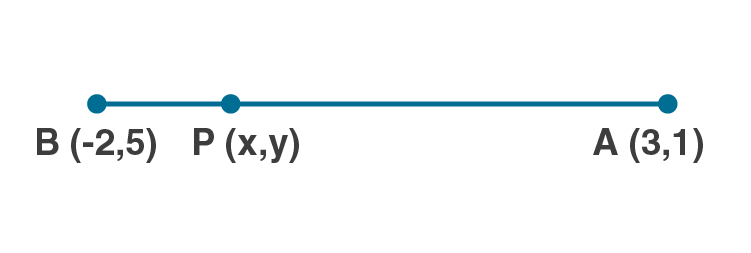# Find the coordinates of the point which is three-fourth of the way from A (3, 1) to B ( – 2, 5).

• 0

An Important Question of M.L Aggarwal book of class 10 Based on Section Formula Chapter for ICSE BOARD.
You have to find the coordinates of the point which is three-fourth of the way from A and B.
This is the Question Number 06, Exercise 11 of M.L Aggarwal.

Share

### 1 Answer

1.Let P be the point which is three-fourth of the way from A(3,1) to B(-2,5).

AP/AB = 3/ 4

AB = AP+PB

AP/AB = AP/(AP+PB) = 3/4

4AP = 3AP+3PB

4AP-3AP = 3PB

AP = 3PB

AP/PB = 3/1

The ratio m:n = 3:1

x1 = 3 , y1 = 1 , x2 = -2, y2 = 5

By Section formula x = (mx2+nx1)/(m+n)

x = (3×-2+1×3)/(3+1)

x = (-6+3)/4

x = -3/4

By Section formula y = (my2+ny1)/(m+n)

y = (3×5+1×1)/(3+1)

y = (15+1)/4

y = 16/4

y = 4

Hence the co-ordinates of P are (-3/4, 4).

• 0